# Regularization method

A method for constructing approximate solutions of ill-posed problems in the following way: As approximate solution of an ill-posed problem (also called an incorrectly-posed problem) one takes the values of a regularizing operator with regard to the approximate nature of the initial data.

For the sake of being specific, consider the problem of finding the solution of a functional equation of the form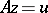, in whichandare elements of metric spacesandwith distance functions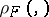and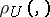, respectively. If, for example,is a completely-continuous operator, then the solution of such an equation need not be stable with respect to small changes of the right-hand side. Suppose that, instead of the exact values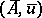of the initial data, approximations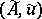are given. In these circumstances one can only ask for approximations to the solution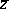of the equation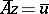. As an approximate solution of an ill-posed problem of this type, with approximate initial data, one cannot take an exact solution of the equation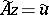, since such a solution need not exist, and even if it does, it need not be stable with respect to small changes of the initial data, so that such a "solution" may not admit a physical interpretation. In what follows, it is assumed for simplicity that only the right-hand sideis approximate, and that the operatoris specified exactly.

Letbe a bound on the deviation of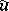from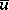, that is, on the distance, and let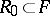be a given class of possible solutions (comparison models). It is natural to search for approximate solutions of the equation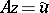among the elementscompatible with the initial data, that is, such that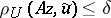. Let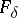be the set of all such elements from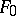. If, in the chosen classof possible solutions, there are no elements (for example, functions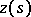) compatible with the initial data, then this means that the elementshave an over-simplified (too rough) structure. In this case it is necessary to extendby taking, if necessary, an increasing sequences of classes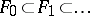until a class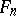is reached that contains elements (for example, functions) that are compatible with the initial data.

Ifis not empty, then it may contain elements (functions) that are essentially different from one another. In such cases, the single requirement of compatibility of a possible solution with the initial data cannot serve as the only criterion for finding a well-defined approximate solution of the equation, as there are insufficient grounds for the choice of an approximate solution from among the compatible elements of.

For stable solutions to be well-defined, one needs a certain principle for selecting solutions compatible with. The formulation of this usually varies in accordance with the nature of the problem. Such a selection can be made, for example, on the principle of choosing an element (functions in) that has minimal complexity. The notion of complexity of an elementcan be formalized, for example, using complexity functionals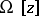— continuous, non-negative functionals satisfying certain special requirements (see ). As a measure of the complexity of an elementone takes the value of the functional. Thus, if the elementsare continuous functionson an intervaland belong to the class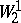, then one can take the complexity functionalto beThe search for approximate solutions of the equationamong the simplest elements (functions) that are compatible with the initial data leads to the problem of finding an element ofthat minimizeson. Ifis a linear operator and ifdoes not have local minima in its domain of definition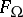, then this problem can be reduced to that (see  for some details) of finding an element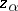in the set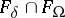that minimizes the functional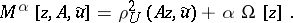The value of the parameter(the regularization parameter) must be chosen in accordance with the level of error in the initial data. For example, it can be defined in terms of discrepancy, that is, by the condition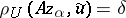, for a given. But there are other ways of determining(see ). Thus,may depend onand,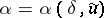. The element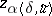is then taken as an approximate solution of the equation. This is also one of the forms of the regularization method developed in , . In a similar way one can formulate approximate solutions of the equationin case both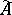andare approximately given. In this case a functional of the typeis minimized (see , for example). There are other possible forms of the regularization method, and applications to other classes of problems (see ). Regularization methods have also been developed for solving non-linear problems (see , ).